Refraction, Snell's law, and total internal reflection

3-20-98

Relevant sections in the book : 26.1 - 26.3

Refraction

When we talk about the speed of light, we're usually talking about the speed of light in a vacuum, which is 3.00 x 108 m/s. When light travels through something else, such as glass, diamond, or plastic, it travels at a different speed. The speed of light in a given material is related to a quantity called the index of refraction, n, which is defined as the ratio of the speed of light in vacuum to the speed of light in the medium:

index of refraction : n = c / v

When light travels from one medium to another, the speed changes, as does the wavelength. The index of refraction can also be stated in terms of wavelength:Although the speed changes and wavelength changes, the frequency of the light will be constant. The frequency, wavelength, and speed are related by: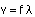The change in speed that occurs when light passes from one medium to another is responsible for the bending of light, or refraction, that takes place at an interface. If light is travelling from medium 1 into medium 2, and angles are measured from the normal to the interface, the angle of transmission of the light into the second medium is related to the angle of incidence by Snell's law :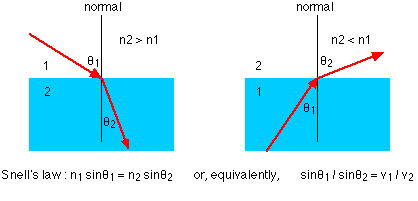When light crosses an interface into a medium with a higher index of refraction, the light bends towards the normal. Conversely, light traveling across an interface from higher n to lower n will bend away from the normal. This has an interesting implication: at some angle, known as the critical angle, light travelling from a medium with higher n to a medium with lower n will be refracted at 90°; in other words, refracted along the interface. If the light hits the interface at any angle larger than this critical angle, it will not pass through to the second medium at all. Instead, all of it will be reflected back into the first medium, a process known as total internal reflection.

Total internal reflection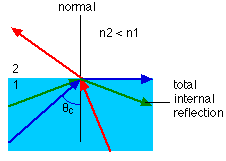The critical angle can be found from Snell's law, putting in an angle of 90° for the angle of the refracted ray. This gives: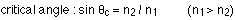For any angle of incidence larger than the critical angle, Snell's law will not be able to be solved for the angle of refraction, because it will show that the refracted angle has a sine larger than 1, which is not possible. In that case all the light is totally reflected off the interface, obeying the law of reflection.

Optical fibers are based entirely on this principle of total internal reflection. An optical fiber is a flexible strand of glass. A fiber optic cable is usually made up of many of these strands, each carrying a signal made up of pulses of laser light. The light travels along the optical fiber, reflecting off the walls of the fiber. With a straight or smoothly bending fiber, the light will hit the wall at an angle higher than the critical angle and will all be reflected back into the fiber. Even though the light undergoes a large number of reflections when traveling along a fiber, no light is lost.

Depth perception

Because light is refracted at interfaces, objects you see across an interface appear to be shifted relative to where they really are. If you look straight down at an object at the bottom of a glass of water, for example, it looks closer to you than it really is. Looking perpendicular to an interface, the apparent depth is related to the actual depth by: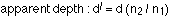An example

A beam of light travels from water into a piece of diamond in the shape of a triangle, as shown in the diagram. Step-by-step, follow the beam until it emerges from the piece of diamond.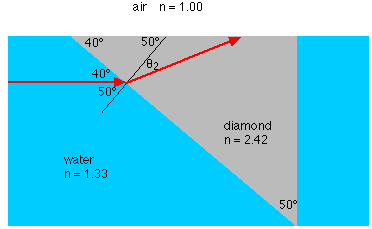(a) How fast is the light traveling inside the piece of diamond?

The speed can be calculated from the index of refraction: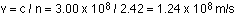(b) What is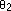, the angle between the normal and the beam of light inside the diamond at the water-diamond interface?

A diagram helps for this. In fact, let's look at the complete diagram of the whole path, and use this for the rest of the questions.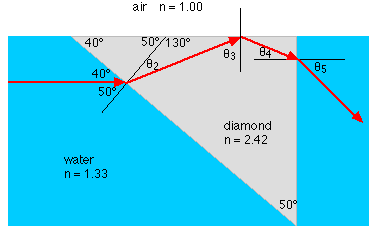The angle we need can be found from Snell's law: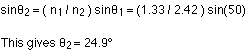(c) The beam travels up to the air-diamond interface. What is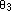, the angle between the normal and the beam of light inside the diamond at the air-diamond interface?

This is found using a bit of geometry. All you need to know is that the sum of the three angles inside a triangle is 180°. Ifis 24.9°, this means that the third angle in that triangle must be 25.1°. So: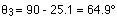(d) What is the critical angle for the diamond-air interface?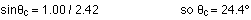(e) What happens to the light at the diamond-air interface?

Because the angle of incidence (64.9°) is larger than the critical angle, the light is totally reflected internally.

(f) The light is reflected off the interface, obeying the law of reflection. It then strikes the diamond-water interface. What happens to it here?

Again, the place to start is by determining the angle of incidence,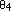. A little geometry shows that: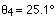The critical angle at this interface is :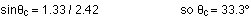Because the angle of incidence is less than the critical angle, the beam will escape from the piece of diamond here. The angle of refraction can be found from Snell's law: